﻿ Physics Laboratory 6

## Physics Laboratory 6

### Simple DC circuits and Resistance Measurements

In this lab you will you will use an on-line simulation from the University of Colorado PhET group to explore simple DC circuits and you will determine the resistance of different resistors by

• reading the code printed onto some of the resistors,
• measuring the resistance using a Wheatstone bridge (simulation),
• calculating the resistance using given properties of the material the resistor is made of.

Open a Microsoft Word document and keep a log of your activities.  Answer all the questions in blue font.

### Part 1, DC circuits

Click the Lab icon.  Explore the interface!

• Components are dragged from the toolbox to make circuits.
• To explore the properties of a component, tap it.  You can then change many properties and also remove the component.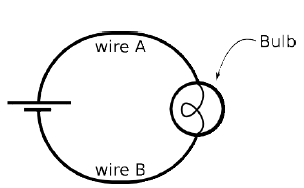(a)  Use one ideal battery (9 V, 0 Ω internal resistance), a light bulb (10 Ω) resistance) and ideal wires (near 0 Ω resistance) to build the circuit shown on the right.  Make sure your light bulb lights up.  Use the voltmeter to measure the potential difference (ΔV) across the battery.  Record only the magnitude of the potential difference (omit +/- signs).  Make a similar potential difference measurement across the bulb and across each length of wire.  With the noncontact ammeter, measure the current through the bulb, IBulb.

Fill in "Table A" below..

ΔVBattery ΔVwire A ΔVwire B ΔVBulb IBulb

Now use two ideal batteries (9 V, 0 Ω internal resistance), three light bulb (10 Ω) resistance) and as many ideal wires as needed to build several different circuits.

(b)  Use all the components (two batteries, 3 light bulbs) and connect them in such to produce the most light.  (The largest possible current should flow through the bulbs.  You can connect the batteries and bulbs in series or in parallel as needed.)

Make measurements and fill in "Table B"  below.

ΔVBattery IBattery ΔVany Bulb Iany Bulb

(c)  Use all the components (two batteries, 3 light bulbs) and connect them in such to produce the least amount of light or current, but not zero light or current.  (The smallest possible non-zero current should flow through the bulbs.)

Make measurements and fill "Table C" below.

ΔVBattery IBattery ΔVany Bulb Iany Bulb

Set up the circuit shown on the right with three 10 Ω bulbs and one 9 V battery.(d)  Observe the brightness of the bulbs.

Make measurements and fill in "Table D" below.

ΔVBattery IBattery ΔVBulb 1 IBulb 1 ΔVBulb 2 IBulb 2 ΔVBulb 3 IBulb 3

(e)  Change the internal resistance of the battery to 1 Ω.  What happens?

Make measurements and fill in "Table E" below.

ΔVBattery IBattery ΔVBulb 1 IBulb 1 ΔVBulb 2 IBulb 2 ΔVBulb 3 IBulb 3

Paste Tables A - E into your log and comment on your measurements.

### Part 2, Resistance Measurements

Background: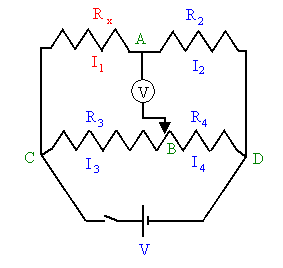Any device that offers resistance to current flow has an equivalent resistance.  If a voltmeter is used to determine the voltage V across the device and at the same time an ammeter is used to measure the current I flowing through the device, then this resistance can be found by dividing V by I, i.e.  R = V/I.

The resistance of the device can also be determined with an ohmmeter.  A simple ohmmeter is a voltage source V in series with an ammeter.  The component, whose resistance is to be measured, is disconnected from any circuit and the ohmmeter is connected across it.  The equivalent resistance is R = V/I, where I is the current flowing through the ammeter.  The resistance of the component is R minus the (usually very small) resistance of the ohmmeter itself.

The accuracy of an ohmmeter is limited by its internal resistance.  When extremely accurate measurements are needed, a Wheatstone bridge is used.  A diagram of a Wheatstone bridge is shown on the right.  A Wheatstone bridge uses four resistances.  R2 is precisely known, it is the reference or standard resistance.  The ratio R3/R4 can be adjusted, but its value is always known.  The diagram shows a single coil that is divided by the tap B.  The ratio of the resistances R3 and R4 equals the ratio of the corresponding lengths of coil.  This device is called a potentiometer.  Rx is the resistance to be determined.  A power supply with a switch is connected across points C and D, and a digital voltmeter is connected across points A and B.

The Wheatstone bridge uses a null measurement to determine the unknown resistance.  When the voltmeter reads zero, the potential at A equals the potential at B.  The bridge is balanced.  When the bridge is balanced, the voltmeter reading does not change when the switch is opened and closed.  Such null measurements are the basis for the most accurate instruments, because, when no current is flowing through the meter, the internal resistance of the meter does not affect the circuit.

If points A and B are at the same potential, then we have

I1Rx = I3R3,
I2R2 = I4R4.

Since no current is flowing through the voltmeter we have

I1 = I2,
I3 = I4.

Therefore we have

Rx/R2 = R3/R4
Rx = R2(R3/R4).

The unknown resistance is determined by reading the ratio R3/R4 of the potentiometer when V = 0.  The dial of a potentiometer displays a number n.  For the potentiometer used in our experiment, n/10 is equal to the ratio R3/(R3 + R4).  We can solve for R3/R4.

R3/R4 = n/(10 - n).

Therefore, when V = 0, we have for the unknown resistance

Rx = R2n/(10 - n).

When a manual refers to a resistor, it usually refers to a device whose only purpose it is to offer resistance to current flow.  The resistance of a resistor is often printed onto the resistor in code.  A pattern of colored rings is used.  Most resistors have three rings to encode the value of the resistance, and one ring to encode the tolerance (uncertainty) in percent.  The colors of the rings are internationally defined to represent integers between 0 and 9.  The integers represented by the different colors are shown in the table below.

 Black Brown Red Orange Yellow Green Blue Violet Gray White 0 1 2 3 4 5 6 7 8 9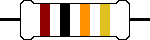• The first band is the band closest to one end of the resistor.  The first band and second band together represent a two-digit integer number.
• Multiply the number represented by the color of the first band by 10 and add the number represented by the color of the second band.  You get a two-digit integer number.
• The number represented by the color of the third band is the number of zeros that must be appended to the number obtained from the first two bands to get the resistance in Ohms.  (If this number is 1, you add one zero, or multiply by 101, if the number is 2, you add two zeros, or multiply by 102, etc.)
• The first ring of the resistor shown above is brown, and the second ring is black.  The two-digit integer number represented by the two rings is 10 + 0 = 10.  The third ring is orange.  Thus 3 zeros must be appended to the number 10, or the number 10 must be multiplied by 103.  The resistance of this resistor therefore is 10000 Ω, or 10 kΩ.
• The next band, (i.e.  the fourth band), is the tolerance band.  The tolerance band is typically either gold or silver.  A gold tolerance band indicates that the actual value will be within 5% of the nominal value.  A silver band indicates 10% tolerance.
• The color of the fourth ring of the resistor shown above is gold.  We expect the actual resistance to be within 5% of the nominal resistance.  i.e.  we expect the actual resistance to lie between 9500 Ω and 10500 Ω.
• If the resistor has one more band past the tolerance band it is a quality band.  Read the number as the % failure rate per 1000 hours, assuming maximum rated power is being dissipated by the resistor.  1% resistors have three bands to read digits to the left of the multiplier.  They have a different temperature coefficient in order to provide the 1% tolerance.

Resistor Color Codes

Color 1st and 2nd
Significant
Figures
Multiplier Tolerance
Black 0 1 --
Brown 1 10 ±1%
Red 2 100 ±2%
Orange 3 1,000 ±3%
Yellow 4 10,000 ±4%
Green 5 100,000 --
Blue 6 1,000,000 --
Violet 7 10,000,000 --
Gray 8 100,000,000 --
White 9 -- --
Gold -- 0.1 ±5%
Silver -- 0.01 ±10%
No Color -- -- ±20%

### Exercise

Find the nominal resistance of three color-coded resistors and the nominal uncertainty in this value.  Record this in table 1 below.

Table 1

Resistors
Nominal R
Ω
Tolerance
%
R1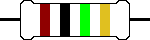R2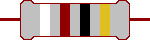R3Series

X
Parallel

X
• Assume the 3 resistors are connected in series.
• Calculate the nominal resistance of the chain and record it in table 1.
• Assume the 3 resistors are connected in parallel.
• Calculate the nominal resistance of this network and record it in table 1.
• Insert Table 1 into your word document.

### Experiment

You have 5 coils of wire, a standard resistance box containing 1-10 ohm precision resistors, a potentiometer, a voltmeter, and a 10 V power supply.  You will measure the resistance of each of the coils with a Wheatstone bridge.  With a switch you can select which standard resistor from the you want to use in the bridge.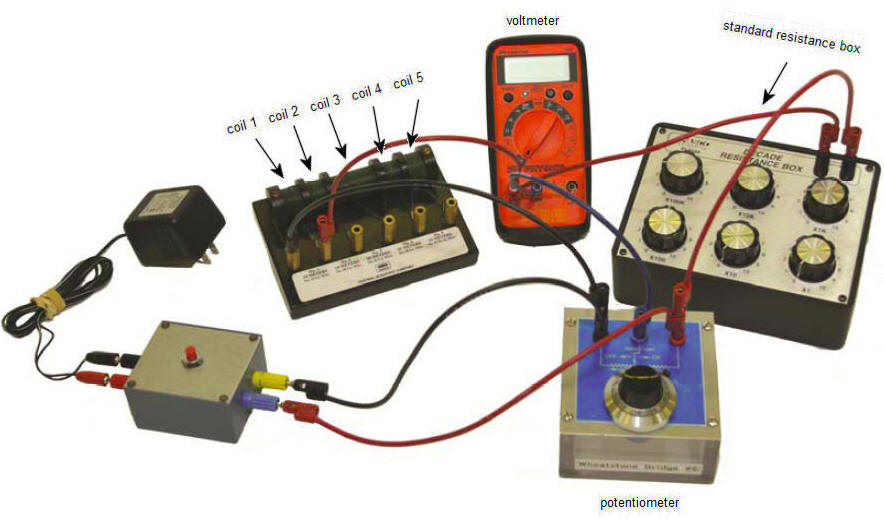Four coils are made of copper wire and one coil is made of nickel silver wire.  The length and the radius of the wire and the resistivity of the material for each coil are listed in the table below.  You will also calculate the resistance of each coil from these given material properties.

Data describing the coils

Coil #
Type
resistivity
(10-8Ωm)
Length L
m
(10-4m)
1 copper 1.7 10 3.2
2 copper 1.7 10 1.6
3 copper 1.7 20 3.2
4 copper 1.7 20 1.6
5 nickel silver 33 10 3.2

A schematic diagram of your Wheatstone bridge circuit is shown below.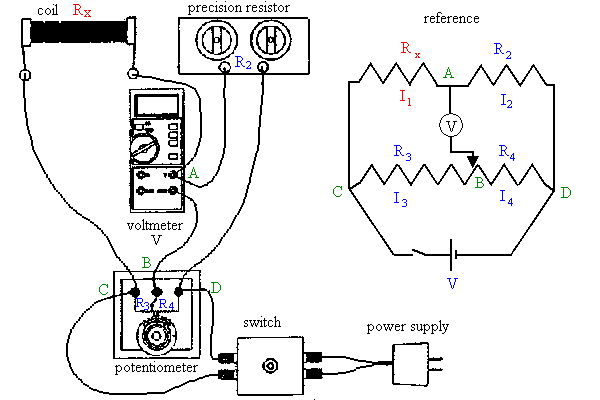• Start the experiment by clicking the link.
Wheatstone Bridge
• Choose a coil and a standard resistance R2 (1 Ω, 3 Ω, or 5 Ω).
In the simulation, close the contact switch (click the OFF/On button) and rotate the potentiometer dial while observing the reading of the digital voltmeter.  Notice that the reading can be positive or negative.  Rotate until you obtain a minimum value close to zero.  (Try to achieve a voltmeter reading of near zero with a potentiometer dial reading between 3 an 7, by selecting an appropriate standard resistance R2.)
• When the Wheatstone bridge is balanced, open and close the switch.  The voltmeter reading should always be close to zero.  Record the reading n of the potentiometer dial, and the value of the standard resistance R2 you selected in the table 2 below for each coil.

Table 2

Coil #
n
R2
Ω
Measured Rx
Ω
Calculated Rx
R = (ρL/A)(Ω)
Difference
%
1
2
3
4
5
• Find the unknown resistance Rx of each coil using Rx = R2n/(10 - n).  Record this value in the table 2 under "Measured Rx".
• Using the data in the table describing the coils, calculate the resistance of each coil.
Use the radius of each wire to compute its cross-sectional area.
Use the length, the cross-sectional area, and the resistivity to calculate and record the resistances of each of the coils of wire.
• Compare the measured and calculated values of the resistances of each of the coils of wire and calculate the percent difference.
• Insert Table 2 into your Word document.

• Make a statement concerning the relationship between the resistance of a wire and its length.  Support your statement by referring to your data.
• Make another statement concerning the relationship between the resistance of a wire and its cross-sectional area.  Extend this statement and relate the resistance of a wire to its diameter or its radius.  Support your statements by referring to your data.
• Is of the following statements true or false?
When a Wheatstone bridge is balanced, no current flows through the resistance being measured.